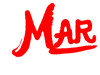# MasterAllRound: the PC calculator

## Linear equation system solver

Solving a system of linear equations means finding those values for the unknowns of the system which satisfy all equations simultaneously. MasterAllRound solves quadratic, linear and single solution equation systems. There is no size restriction for MasterAllRound.

#### Cell editing

As with the matrix modules you have several options to edit the cells:
• You can enter numbers. For example "2.55"
• You can enter formulas. For example "1.5 * (5/7 + 3/8)^2"
• You can work with number symbols

#### Example

Let's assume that we have to solve the following problem:
• Mr. Smith buys 5 apples, 5 pears and 4 cucumbers. He pays 3.30 EUR
• Ms. Miller buys 2 apples, 3 pears and 4 cucumbers. She pays 2.10 EUR
• Mrs. Taylor buys 1 apples, 1 pears and 1 cucumbers. She pays 0.70 EUR
The question is: what is the cost of each apple, pear and cucumber? We can describe the problem by:
• 5 apples + 5 pears + 4 cucumbers = 3.30 EUR
• 2 apples + 3 pears + 4 cucumbers = 2.10 EUR
• 1 apples + 1 pears + 1 cucumbers = 0.70 EUR
or, using x1 for apple, x2 for pear und x3 for cucumber:
• 5 x1 + 5 x2 + 4 x3 = 3.30
• 2 x1 + 3 x2 + 4 x3 = 2.10
• 1 x1 + 1 x2 + 1 x3 = 0.70

In matrix formulation the problem is written as: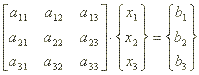or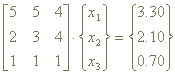respectively.

The augmented matrix is then:The calculation with MasterAllRound is easy and straightforward:

• Enter the number of equations, in our examples "3"
• Enter the augmented matrix, i. e. the coefficients of the matrix and the column vector
• Click on the "Run!" button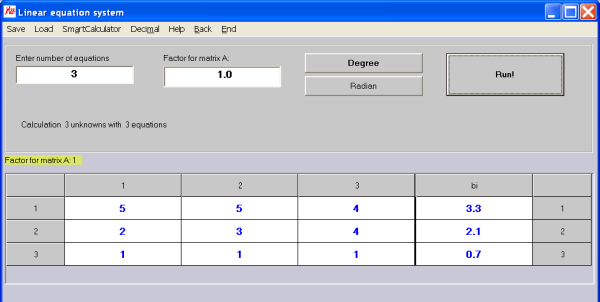The result window pops up: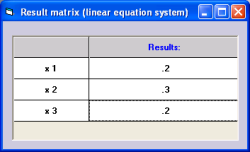You would obtain the result: x1 = 0.2; x2 = 0.3; x3 = 0.2.
That is: 1 apple costs 0.20 EUR, 1 pear costs 0.30 EUR and 1 cucumber costs 0.20 EUR.

#### Application examples

With MAR Plus you can easily and quickly obtain results for linear equation systems. Linear equation systems are commonly used in many professional areas. Here's an example of how to use a gozintograph in material management and the solution of the resulting linear equation system with MAR Plus.